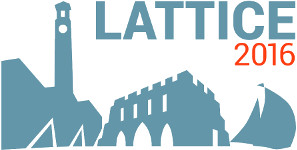#The 34th International Symposium on Lattice Field Theory (Lattice 2016)

Jul 24 – 30, 2016
Highfield Campus, University of Southampton
Europe/London timezone

## Quark masses and strong coupling constant with Highly-Improved Staggered Quarks

Jul 26, 2016, 2:00 PM
20m
Building 67 Room 1007 (Highfield Campus, University of Southampton)

### Building 67 Room 1007

#### Highfield Campus, University of Southampton

Highfield Campus, Southampton SO17 1BJ, UK
Talk Standard Model Parameters and Renormalization

### Speaker

Dr Yu Maezawa (YITP, Kyoto University)

### Description

We present new results on the charm and bottom quark masses as well as the strong coupling constant in 2+1-flavor QCD using Highly Improved Staggered Quark action and gauge configurations generated by HotQCD Collaboration. Our approach is based on calculating the moments of meson correlators and we use a wide range of lattice spacing up to $a^{-1} \sim 4.9$ GeV in our study. The ratios of quark masses $m_c/m_s$ and $m_b/m_c$ are obtained from the combinations of the pseudo-scalar and vector meson masses and found to be $m_c/m_s=11.871(96)$ and $m_b/m_c=4.528(57)$ in the continuum limit. The lattice results of the hyper-fine splitting of the charmonium can reproduce the experimental value in the continuum. We also perform the determination of the strong coupling constant in the $\overline{\rm MS}$ scheme from the moments of pseudo-scalar charmonium correlators and find $\alpha_s(\mu=m_c)=0.3697(56)$, which is the determination of $\alpha_s$ at lowest energy scale so far. For the charm quark mass we obtain $m_c(\mu=m_c)=1.2668(10)$ GeV.

### Primary author

Dr Yu Maezawa (YITP, Kyoto University)

### Co-author

Dr Peter Petreczky (Brookhaven National Laboratory)

 Slides